Yang J-S, Nam H-J, Seo M, Han SK, Choi Y, et al. 2011 OASIS: Online Application for the Survival Analysis of Lifespan Assays Performed in Aging Research. PLoS ONE 6(8): e23525. Please use this paper to cite OASIS.
 Submission  | Introduction  | Recent changes  | Contact

## Summary

Online application for survival analysis (OASIS) is a one-stop tool for various statistical tasks involved in analyzing survival data in a user-friendly manner.

OASIS provides a uniform platform that is an essential application to facilitate efficient statistical analyses of survival data in the ageing field.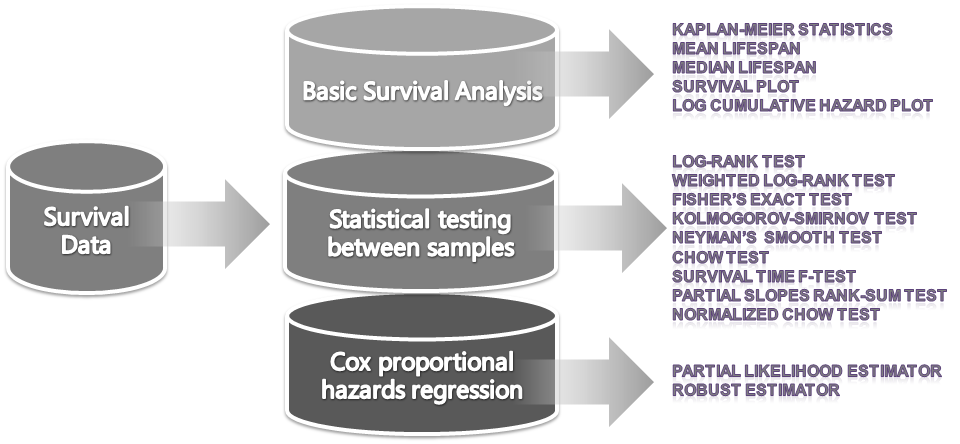The statistical features of OASIS include the calculation of Kaplan-Meier estimates, mean/median lifespan, mortality rate, Mantel-Cox Log-Rank test, Fisher's exact test, weighted Log-Rank test, Kolmogorov-Smirnov test and Neyman's smooth test.

Moreover, OASIS generates survival and mortality curves that can be easily exported and modified by using common graphic softwares.

We provides OASIS source codes for statistical analyses of survival data. All codes are provided as open source under a GNU General Public License (GPL).

### Basic Survival Analysis

Python (higher than 2.4) and matplotlib should be properly installed. [ Download ]

### Statistical testing methods

Python (higher than 2.4), numpy, rpy, statlib, chow test, R, and surv2sample (R package) should be properly installed. [ Download ]

### Cox proportional hazards regression

Python (higher than 2.4), rpy, R, and coxrobust (R package) should be properly installed. [ Download ]

## Basic survival analysis

### Input Format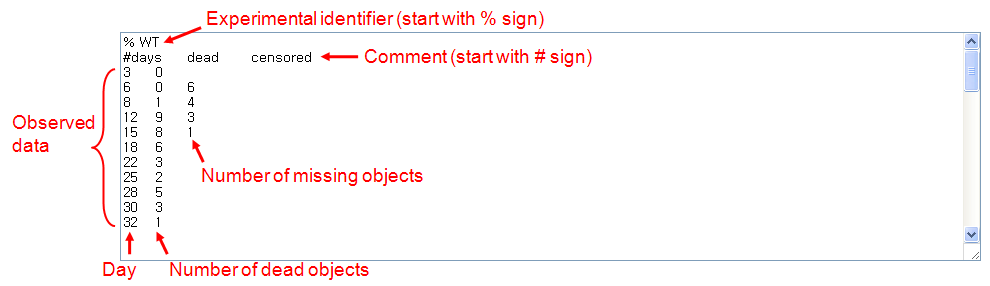As shown in above figure, OASIS takes following format of input data for basic analysis and statistical testing between samples. The data should be tab-delimited.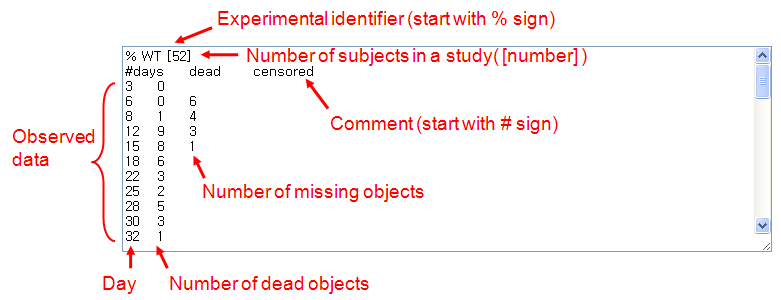% Condition Identifier (Required) [Total number of subjects] (Optional)

# Comment (Optional)

Survival Data (Required, The data should be Tab-Delimited)
• First column should be time (Required)

• Second column should be the number of deaths (Required)

• Other columns are the number of censored data withe different reasons (At least one column is required)

### Output Format

In basic survival analysis, OASIS provides several survival statistics such as Kaplan-Meier estimator, Mean/Median lifespan, Survival curve and Mortality curves which can help to user interpret their survival data.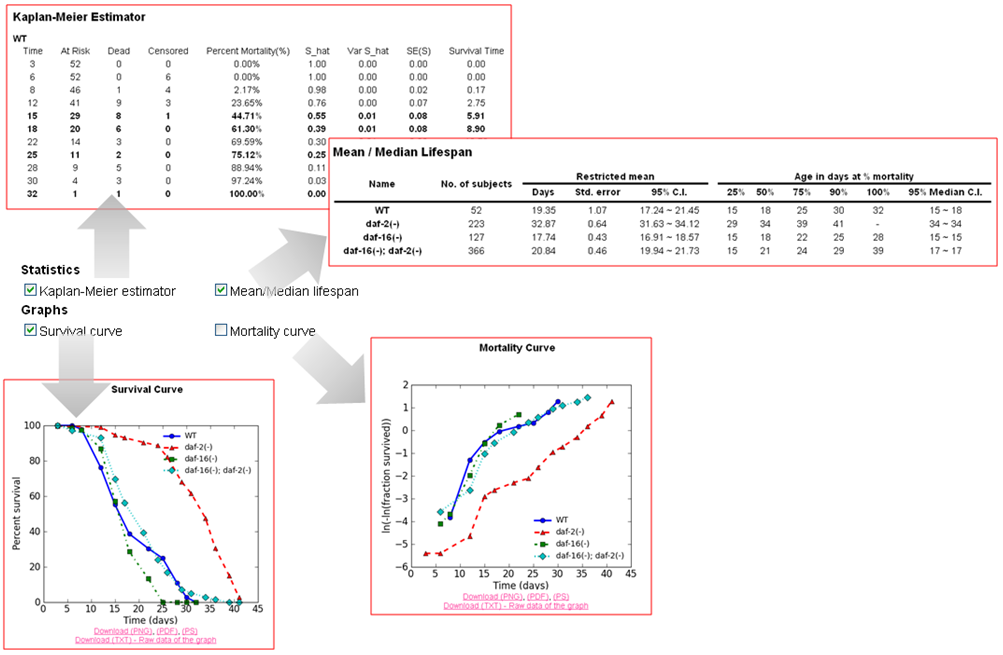### Statistics for the estimation of observed survival

In ageing research, a description of survival data such as the estimation of mean lifespan is essential for determining the effects of a drug treatment or genetic manipulation on ageing. Thus, one of the primary objectives of survival analysis is the estimation of survival function from incomplete datasets. To estimate survival time as the area under the survival curve, it is necessary to characterize the survival function which is a probability of death after some specific time t.

• 1. Kaplan-Meier estimator

The Kaplan-Meier estimator estimates the survival or the hazard function from observation of lifetimes. It is kind of explanatory method for the time to event, where the time is considered as the most prominent variable. In lifespan studies, it might be used to measure the fraction of organisms living for a certain amount of time after event. To estimate survival time as the area under the survival curve, it is necessary to characterize the survival function which is a probability of death after some specific time t.where t is some specific time, T is a random variable of the time, and P denotes the probability of death. Generally, we only observe censored data which include missing subjects. The Kaplan-Meier or product-limit estimator was proposed for right censored analysis and it is the most common method of estimating the survival function S(t).where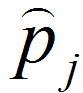is the conditional probability of surviving during the jth interval, dj is the number of deaths and ni is the size of the population at risk during the jth interval. The variance of Kaplan-Meier estimator at time point ti can be estimated by Greenwood's formula. Although the calculated variance tends to underestimate the true variance of Kaplan-Meier estimator for small to moderate-sized samples, it is relatively close to true variance and therefore commonly used in various studies.whereis the Kaplan-Meier estimator, dj is the number of deaths and ni is the size of the population at risk during the jth interval. Standard error of Kaplan-Meier estimator was given by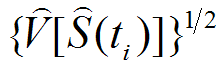• 2. Mean lifespan

Mean survival time is estimated as the area under the survival curve. The estimator is based upon the entire range of data. The Irwin's restricted mean lifespan, which was described by Kaplan and Meier as well, was estimated as the area under the survival curve by the following formula.whereis the largest observed time andis estimated with Kaplan-Meier method.

• 3. Lifespan at % mortality

The ratio of total deaths to total population over specified period time. For example, a mortality rate of 10.5 in a population of 1,000,000 would mean 1050 deaths per unit time

• 4. Survival plot

A graph that shows the relationship between the survival and age of a population of a particular species, plotting the number or population of organisms surviving at each age for a given group. The Kaplan-Meier or product-limit estimator was proposed for right censored analysis and it is the most common method of estimating the survival function S(t).whereis the conditional probability of surviving during the jth interval, dj is the number of deaths and ni is the size of the population at risk during the jth interval.

• 5. Log cumulative hazard plot

The cumulative hazard function (H), which is the risk of death at time t, was estimated by following formula.whereis the Kaplan-Meier estimator.
Mortality curve shows the change in mortality rates over specified period time, plotting the number of deaths at each age for a given group.
Please note that the analysis of age-specific mortality rate by using this graph can cause biased results when the sample size is not sufficiently large (Promislow et al., 1999). Users should be cautious about interpreting the results if the survival data were obtained from small samples sizes.

• ## Statistical testing between samples

### Input Format

Input Format for statistical testing is same as that of basic survival analysis

### Ouput Format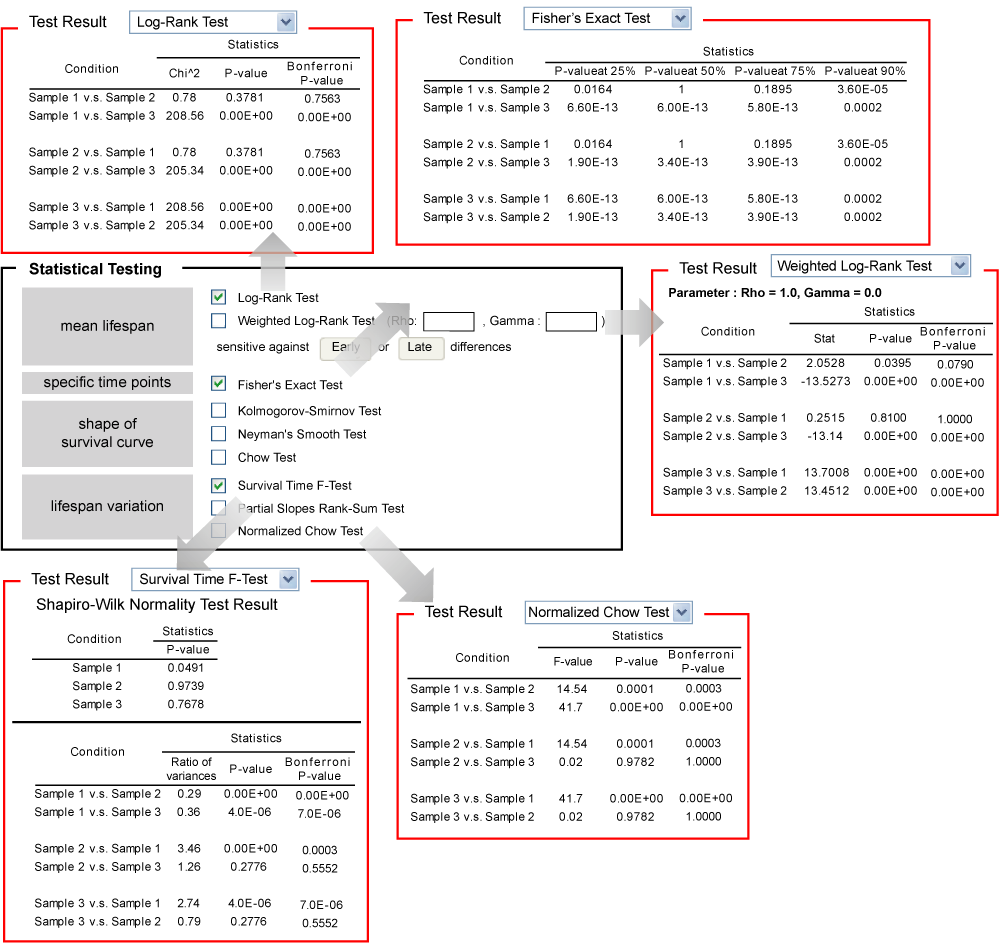### Test for the significance of difference in lifespan

In a lifespan study, the comparisons of survival functions between experiment and control groups are important to determine the efficacy of the experimental treatments such as genetic manipulation, dietary intervention, or drug treatments. To systematically compare survival functions between experiment and control, we need to check various statistics in survival datasets because different conditions may increase or decrease lifespan in different ways. For example, some conditions could only increase the average lifespan, whereas others could increase both of average and maximum lifespan. Therefore, the statistics of overall lifespan is compared using log-rank test, whilst those of a specific time point is compared with Fisher's exact test. Based on comparisons of various statistics with overall lifespan, we can infer which condition reduces mortality caused by mid-life diseases or slow down fundamental processes of ageing.

• 1. Log-rank test

Mantel-Cox test, so-called log-rank test, is a kind of nonparametric test which is frequently used for the comparison of two survival functions through overall lifespan data. The log-rank statistics in two groups such as experiment and control is calculated as follows.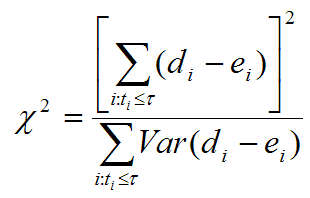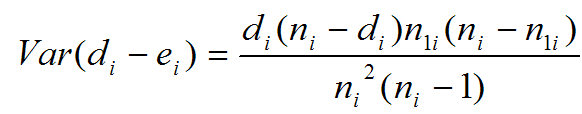where di is the number of deaths in group 1, and ei (estimated as (dinli)/ni ) is the number of expected deaths in group 1. n1i is the size of the population of group 1 at risk during the ith interval, and ni is the total size of population at risk during the ith interval.
P-value 0.00E+00 is provided when P < 1.0 * 10-10.
• 2. Weighted log-rank test

One might want to put more emphasis on earlier deaths than the later ones or vice versa. To generalize log-rank test for these needs, Fleming and Harrington developed G(rho, gamma)-weighted log-rank test. The weighted test statistics is calculated by following equation.where wi represents the G(rho, gamma) weight defined as S(t)rho(1-S(t))gamma. Generally if rho > 0 and gamma = 0, the test is sensitive against early difference, whereas if rho = 0 and gamma > 0, the test is sensitive against later differences.
P-value 0.00E+00 is provided when P < 1.0 * 10-10.
• 3. Fisher's exact test

Fisher's exact test is frequently used in survival analysis. To test different survival functions at specific time point instead of overall lifespan, the program can calculate the probability of observed data with Fisher's exact test at different time points as following formula.where a and b are the number of living subjects in group 1 and group 2 respectively and c and d are that of dead subjects in group 1 and group 2 respectively at specific time t. P-value of Fisher's exact test was calculated with the sum of probabilities less than or equal to pt of all combinations. Generally, 90% mortality is used for Fisher's exact test. However, in some cases, comparisons between two datasets showed no statistically significant difference because of several reasons, including drastic death at old age. This analysis suggests that one might want to put more emphasis on earlier deaths than the later ones because later deaths might result from causes unrelated to normal ageing.

• 4. Kolmogorov-Smirnov test

While the log-rank test is commonly used for comparing survival data between samples, it is optimized for special assumptions on the underlying distributions such that the hazard ratio or relative risk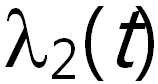/is constant in time t. In that case, a log-rank test generally gives optimal results. However, for considering general situation, a generalized test that does not depend on a special underlying distribution is needed. The Kolmogorov-Smirnov test is an appropriate solution for this purpose so that it robustly works in the condition where the hazard functionsandcross over through time t. The Kolmogorov-Smirnov test is based on the following equation.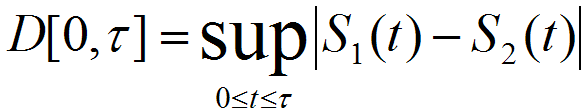where sup represents a supremum of a set which gives smallest real number that is greater than or equal to every number in the set and D represents the largest absolute vertical deviation. OASIS adopted surv2.ks function implemented in R packages (R Development Core Team and contributors worldwide, 2008) to provide Kolmogorov-Smirnov test. We note that the Kolmogorov-Smirnov test in OASIS is not applicable to survival data that contain tied observations [eg. multiple events (death or failure) during an observed time interval]. OASIS provides a warning message if there is any tied observation in survival data within or between samples.
• 5. Neyman's smooth test

Another test capable of detecting a wide spectrum of alternatives is Neyman's smooth test. It is developed to test the homogeneity of two different survival data by comparing a null model, S1(t) = S2(t), and various alternative models. The alternative models embedded the null model with Legendre polynomials based on Neyman's goodness-of-fit idea as following equation.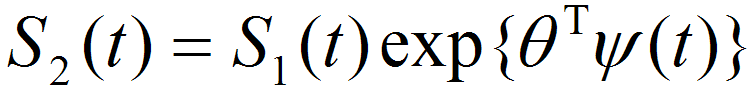where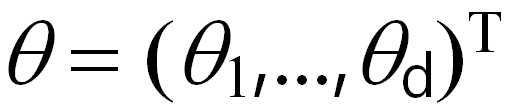is a parameter set of bounded functions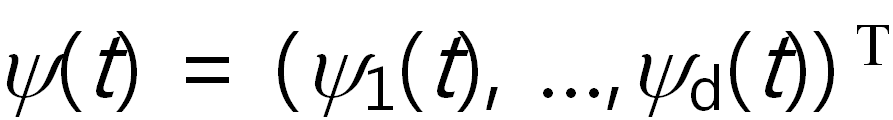which are modelling possible difference between S1(t) and S2(t). Therefore, if, then null hypothesis is accepted. Since the Neyman's smooth test selects optimal smooth model in Legendre polynomials with Schwarz's selection rule, it is different from Kolmogorov-Smirnov test in the respect of providing an idea of the types of difference between two survival data. The selected dimension represents type of difference between S1(t) and S2(t). If the selected dimension (d) is 1, it suggests that S1(t) and S2(t) are different from each other by the constant hazard ratio. If the selected d is 2, then the relationship between two samples is likely to be monotonic. If the selected d is 3, then relationship between two samples is likely to have convex or concave form. OASIS adopted surv2.neyman function implemented in R packages to provide the Neyman's smooth test. Similar to the Kolmogorov-Smirnov test, the Neyman's smooth test is not currently applicable when there are tied observations in survival data.
• 6. Chow test

Chow test, a variant of F-test, was invented by economist Gregory Chow to test whether the coefficients in two linear regressions on different data sets are same or not. This test is generally used for detecting structural break that is an unexpected shift in time series data. In the OASIS, we used this analysis for detecting structural differences between two different log cumulative hazard functions by using the following equation.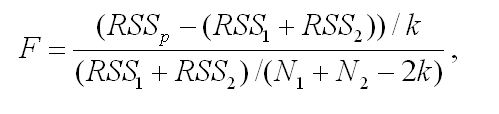where RSSp represents the sum of squared residuals from the pooled log cumulative hazard data. RSS1 and RSS2 represent the sum of squared residuals from two different log cumulative hazard data respectively. N1 and N2 are the number of observation in each data and k is 3 which is the total number of parameters of linear regression model. The test statistic follows the F distribution with k and N1 + N2 - 2k degrees of freedom. OASIS adopted chow.py function implemented by Dr. Ernesto P. Adorio in http://adorio-research.org/wordpress/?p=1789.
• 7. Survival time F-test

We provide survival time F-test, which is used to examine whether two normal populations have same variance or not. Because censored data are generally used in survival analysis, one can estimate the number of dead animals using survival function S(t) and then perform F-test for the comparison of variance between two different survival data.
The F-test is used under the condition that the survival times of individual follow normal distribution. As a normality check method for the given dataset, we provide the Shapiro-Wilk test in the OASIS website. If a P-value from the Shapiro-Wilk test is smaller than 0.01, then the chance of survival data following the normal distribution is less than 0.01, and therefore the results of F-test are not applicable. Thus, we provide warning message in this case.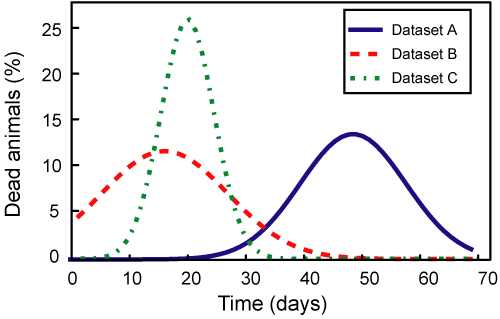• 8. Partial slopes Ranksum test

We devised another statistical test method for comparing the difference of slope of two log cumulative hazard plots. We calculated partial slopes of the log cumulative hazard plot. With null hypothesis that two different log cumulative hazard plots have same slopes, we conducted rank-sum tests with set of partial slopes as following definitions.
The partial slopes rank-sum test is based on non-parametric statistics that requires sufficient number of samples (in this case, partial slopes) for the reliable analysis. Since a partial slope is defined as the changes in log cumulative hazard divided by the corresponding change in survival time between two neighbouring time points, the number of observed time points rather than the total sample size is important for the non-parametric analysis. To obtain statistically meaningful results, at least six observed time points are needed.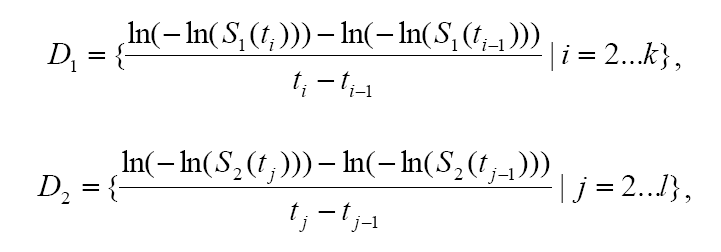,where D1 and D2 are set of partial slopes of each group. These sets are compared with ranksum test.
• 9. Normalized Chow test

Chow test is used for testing whether the coefficients in two linear regressions on different datasets are same or not. However, researchers who perform survival analysis tend to be interested in examining the difference in slope rather than in determining the difference in y-intersect. For this purpose, before conducting Chow test, we normalized the log cumulative hazard data to have a mean of zero. In this case, the linear regression of each dataset has zero y-intersect. Thus, one can test the difference of the slope of each dataset and the pooled data.
We verified the difference of lifespan variations through normalized Chow test, a statistical test that examines whether the coefficients of two linear regressions on different normalized data sets are equal. Like log-rank test, the assumption is that survival rate is constant over time to apply the normalized Chow test.

• ## Cox proportional hazards regression

### Input FormatOASIS takes following format of input data for Cox proportional hazards regression.

% Name of fields (Required)

Survival Data (Required, The data should be Tab-Delimited)
• First column should be time (Required)

• Second column should be the status of events (Required)

• Other columns are considered as the values of risk factors (At least one column is required)

### Ouput Format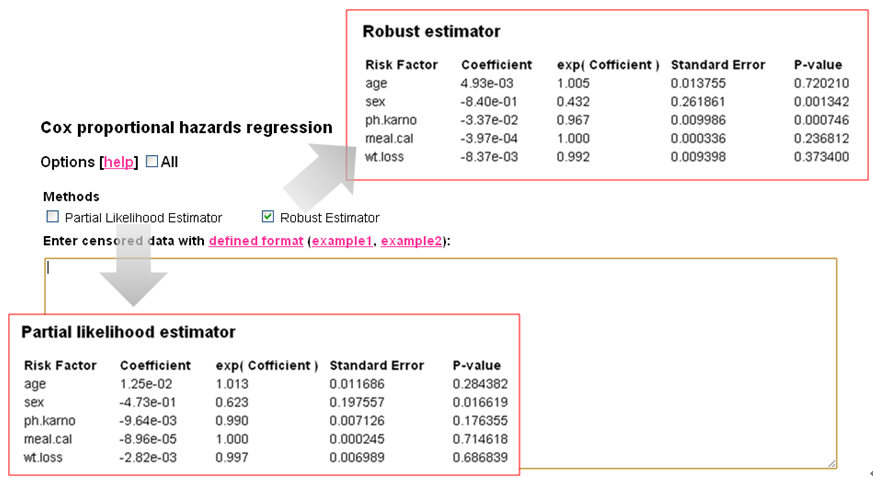### Evaluating the effect of several risk factors

OASIS provides Cox proportional hazards regression which can evaluate the effect of several risk factors such as sex, age, and weight on survival on survival. By considering that hazard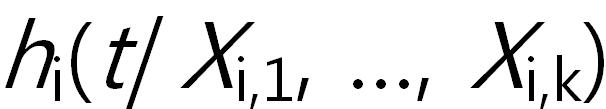function such as mortality rate can be explained by the proportional sum of risk factors, Cox formulated semi-parametric model with following equation.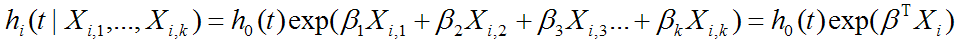whererepresent k risk factors which are assumed to act independently,are their regression coefficients, h0(t) is the baseline hazard at time t, and i is a subscript for observation. To find risk factors that can explain hazard function with proportion, the input data format should be different from that of survival analysis. Therefore, we made a separate input form for Cox proportional hazards regression.

• 1. Partial likelihood estimator

OASIS provides two kinds of regression methods using partial likelihood estimator (PLE) and robust estimator through coxr function in R package. The PLE is generally used to estimate the regression coefficients by maximizing the partial likelihood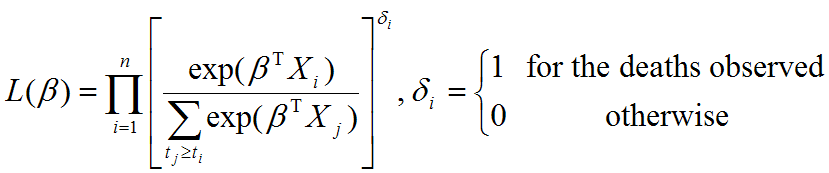whereis a k x 1 vector of regression coefficients, Xi is a k x 1 vector of risk factors, and ti is a observed time of event. To estimate, which maximizes the partial likelihood, Cox's estimator solves the following score function that is the derivative with respect toof the log partial likelihood function.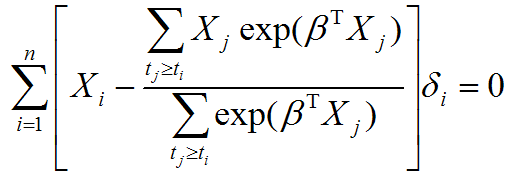where ti is a observed time of event andequals 1 for the deaths observed and 0 otherwise.
• 2. Robust estimator

While the PLE is commonly used for Cox proportional hazards regression, it is known to be sensitive to outliers and the deflection of underlying assumption. Especially the PLE method is strongly influenced by large values of t exp(TX). To reduce the influence of large values of t exp(TX), Minder and Bednarski introduced smooth modification to the score function of the partial likelihood method,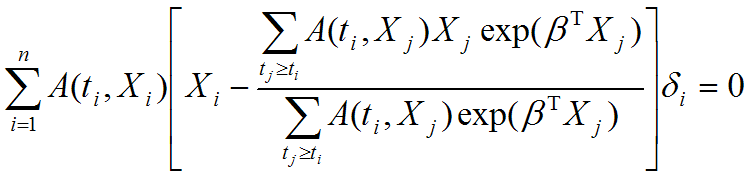where smooth function A(ti, Xi) is defined as A(ti, Xi) = M-min( M, ti exp(TXi)) and M is a 95th percentile of the samples {t1 exp(TX1), ..., tn exp(TXn)}. The smooth functions in the outer and inside sum have an reducing effect on large values of t exp(TX) andTX respectively. OASIS adopted coxr function implemented in R packages (R Development Core Team and contributors worldwide, 2009) to provide the robust estimation. In the coxr function, a better solution for smooth function A(ti, Xi) is used by using(t)exp(TX) instead of heuristic t exp(TX), where(t) is -logS(t).

• # References

 Description Reference Url Kaplan-Meier Estimator - http://en.wikipedia.org/wiki/Kaplan-Meier_estimator Survival Function - http://en.wikipedia.org/wiki/Survival_function Examples - http://www.weibull.com/LifeDataWeb/nonparametric_analysis.htm Examples - http://www.statsdirect.com/help/survival_analysis/kaplan.htm Wilcoxon signed-rank test - http://faculty.vassar.edu/lowry/wilcoxon.html Wilcoxon rank sum test - http://elegans.swmed.edu/~leon/stats/utest.html Fisher's exact test - http://www.socr.ucla.edu/htmls/ana/FishersExactTest_Analysis.html Fisher's exact test - http://faculty.vassar.edu/lowry/fisher.html Fisher's exact test - http://www.langsrud.com/fisher.htm Log Rank Test - http://bioinf.wehi.edu.au/software/russell/logrank/index.html Kaplan-Meier survival function - http://www.hutchon.net/Kaplan-Meier.htm SURVSOFT Geiss K et al.,2009 http://www.krebsregister-bayern.de/software_e.html coxrobust Bednarski T et al., 2006 http://cran.r-project.org/web/packages/coxrobust/index.html Chow test Dr. Ernesto P. Adorio http://adorio-research.org/wordpress/?p=1789

Promislow DEL, Tatar M, Pletcher SD, Carey JR (1999) Below-threshold mortality: implications for studies in evolution, ecology and demography. J. Evol. Biol. 12, 314-328.